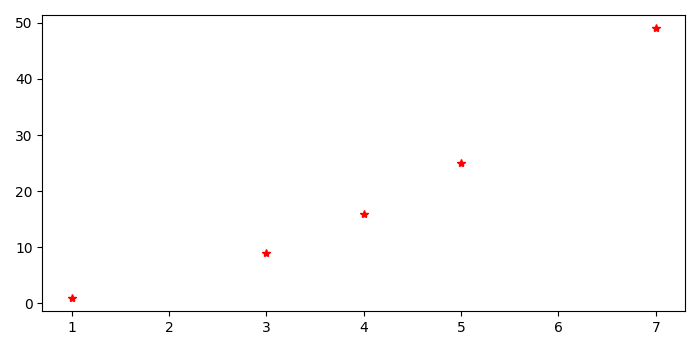# How do I plot a step function with Matplotlib in Python?

To plot a step function with matplotlib in Python, we can take the following steps −

• Create data points for x and y.

• Make a step plot using step() method.

• To display the figure, use show() method.

## Example

import matplotlib.pyplot as plt
import numpy as np
plt.rcParams["figure.figsize"] = [7.00, 3.50]
plt.rcParams["figure.autolayout"] = True
x = np.array([1, 3, 4, 5, 7])
y = np.array([1, 9, 16, 25, 49])
plt.step(x, y, 'r*')
plt.show()

## Output